# Exponent Properties on the GMAT

Because of their clarity and concision, the laws of exponents lend themselves well to GMAT math, especially to the Data Sufficiency format.  If math isn’t your thing, then perhaps the last time you gave any thought to exponents was back in Algebra Two, and perhaps exponents weren’t your favorites there either.  Take heart!  In this post, I will explain the properties you need to know to be successful on the GMAT Quantitative section.

## What is an Exponent?

Fundamentally, an exponent is how many times you multiply a number, that is, how many factors of a number you have.  This is the fundamental definition.  The expression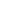means: multiply four 5’s together.  The expressionmeans: multiply three 2’s together, which gives an answer of 8, so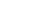.  Technically, 2 is the “base”, 3 is the “exponent”, and 8 is the “power.”  The action of raising something to an exponent is called “exponentiation.”

## Distribution

Just as multiplication distributes over addition & subtraction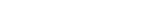so exponentiation distributes over multiplication and division.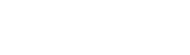Why is that?  Well, consider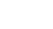.  This means the thing in parentheses multiplied by itself three times: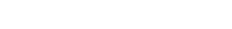.  Well, when we have a bunch of factors, we can rearrange them in any order, because order doesn’t matter in multiplication.  So, I could rearrange them as follows: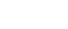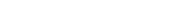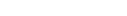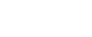All the laws of exponents make sense if you just go back to the fundamental definition.

In this context, I will say: beware of one of the most tempting mistakes in all of mathematics.  Exponentiation does NOT distribute over addition.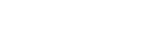Beware.  Even when you know this is wrong, even when you make an effort to remember that it’s wrong, the inherent pattern-matching machinery of your brain will automatically pull your mind back in the direction of making this mistake.  You must be vigilant to avoid this mistake.

## Multiplying Powers

What happens when you multiply two unequal powers of the same base?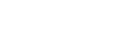Well, let’s think about a concrete example.  Suppose we are multiplying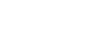.  Well, by the fundamental definition,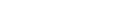, and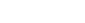, so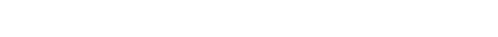If we start with five factors of x, and “stir in” three more factors of x, we wind up with a total of eight factors.  All we have to do is add the exponents.  We can simply generalize this pattern: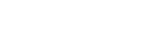Don’t just memorize this: make sure you understand the logic that leads to it.  Remembering with the logic is 100x more effective than blind memorization!

## Dividing Powers

What happens when you divide two unequal powers of the same base?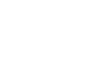As with last time, a concrete example will illuminate the question.  Suppose we divide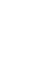.  By the fundamental definition,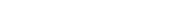and, soIf we start out with seven factors, and then cancel away three of them, we are left with four.  We just subtract the exponents.  We can also generalize this pattern: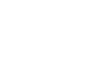Once again: understand the logic, because remember through understanding is considerably more powerful than blind memorization.

## An Exponent of Zero

Mathematicians love to extend patterns.  One example of this is the zero exponent.  If we just see x^0, we may wonder: how on earth are we going to understand what this could mean?  We are clearly outside of the realm where the fundamental definition helps us.

One clever trick we can us is to employ the pattern found in division of powers.  Suppose we have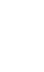— then, the “subtraction of exponents” would imply:but just fundamental logic would tell us that anything over itself equals one.  Therefore, this expressionmust have a value of 1.  That, in turn, tells us the value of.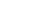.

## Negative Exponents

Here, we will extend the patterns even further.  Consider this chart, for a base of 2:

 Exponent 0 1 2 3 4 Power 1 2 4 8 16

Each time we move one cell to the right, the power gets multiplied by 2, and each time we move one cell to the left, the power gets divided by 2.  That’s a very easy pattern to extend to the left:

 Exponent –4 –3 –2 –1 0 1 2 3 4 Power 1/16 1/8 1/4 1/2 1 2 4 8 16

All we have done was to extend the pattern “move one cell to the left, and the power gets divided by 2.”  The result, we see, is that negative powers are reciprocals of their corresponding positive powers.  This is consistent with the Division of Powers rule: if dividing means subtract the exponents, then an exponent of –3 means we are dividing by three factors of the number.  Therefore, the general rule is:## Summary

I can’t urge enough: the key to remembering these rules is understanding the logic of the arguments behind them.  If you understand these rules, you will understand whatever the GMAT throws at you concerning exponents.

Here are some practice questions:

## Author

•Mike served as a GMAT Expert at Magoosh, helping create hundreds of lesson videos and practice questions to help guide GMAT students to success. He was also featured as "member of the month" for over two years at GMAT Club. Mike holds an A.B. in Physics (graduating magna cum laude) and an M.T.S. in Religions of the World, both from Harvard. Beyond standardized testing, Mike has over 20 years of both private and public high school teaching experience specializing in math and physics. In his free time, Mike likes smashing foosballs into orbit, and despite having no obvious cranial deficiency, he insists on rooting for the NY Mets. Learn more about the GMAT through Mike's Youtube video explanations and resources like What is a Good GMAT Score? and the GMAT Diagnostic Test.